# Dependence of Rate of Reaction in CHEMICAL KINETICS, an innovative topic of Physical Chemistry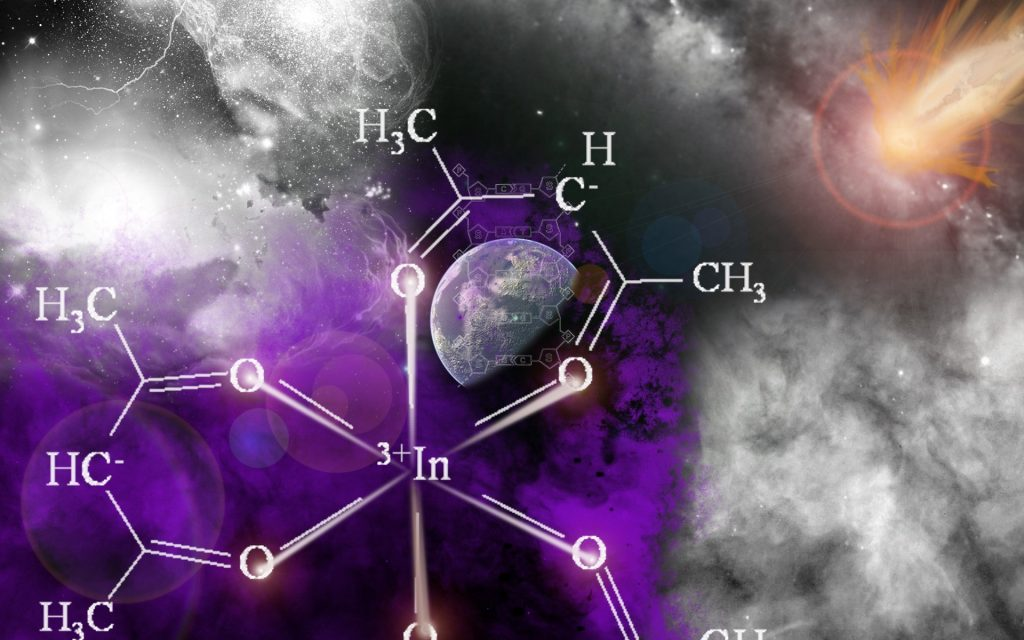## Dependence of Reaction Rates on concentration (Rate law expression)

When a chemical reaction proceeds, concentration of reactant decreases while that of products increases.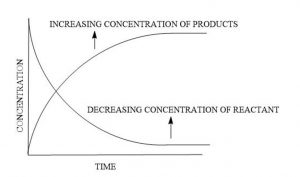→ It is indicated from the fig, In the biginning, only reactants are present so that the concentration of product is Zero
→As the reaction proceeds, the concentration of the reactants decreases and that of preoduct increases.
→At the start of the reactant, rate of reaction is large and it decrease with passage of time. It means that rate of reactant is directly proportional to concentration of reactants. To increase the rate of a reactant we should increase the concentration of one or all reactants and vice.versa.
→In the gas phase, the increse in pressure of the reactant gases increase in pressure, the number of molecule per unit valume increase and therefore, rate of reactant increase.

## Law of Mass Action

→The quantitave relationship between the rate of a reaction and the molar concentration of the reacting substance was given by Norwegian Chemists, Guldbege and waage. This relationship is called Law of mass action
→According to his law; At a given temperature the rate of chemical reactant is directly proportional to the product of the molar concentration of the reactants. The molar concentration of reactants is also called active mass.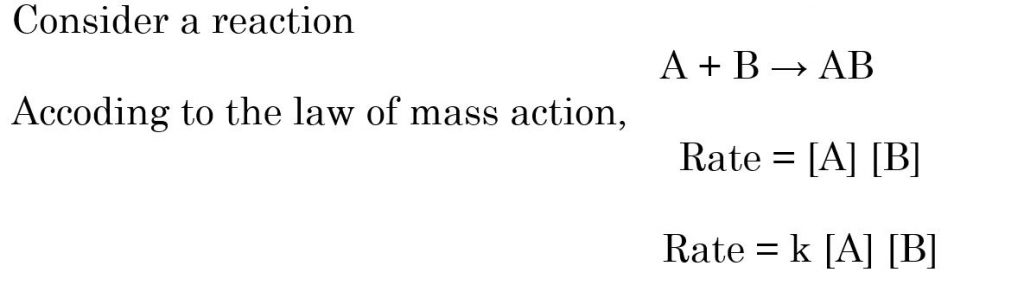where [A] and [B] are molar concentration of [A] and [B]
K → Proportionality constant, called rate contant, also called velocity constant
If [A] = [B] = 1 (unity) then rate = K
Thus rate constant of a reactnat at given temperture may be defined a rate of reaction when concentration of each of the reactant is taken as unity

### Characteristic of a Rate constant

The rate constant has the following properties-
(i) Rate constant is a measure of rate of reaction
Larger the value of K , Faster is the raection
Smaller the value of K , Slower is the reaction
(ii) Different reactnt have different value of K
(iii) At a fixed temperture, the value of K constant and is characteristic of the reaction. However, it changes with temperature
For the particular order reactant, the rate constant is independent of concentration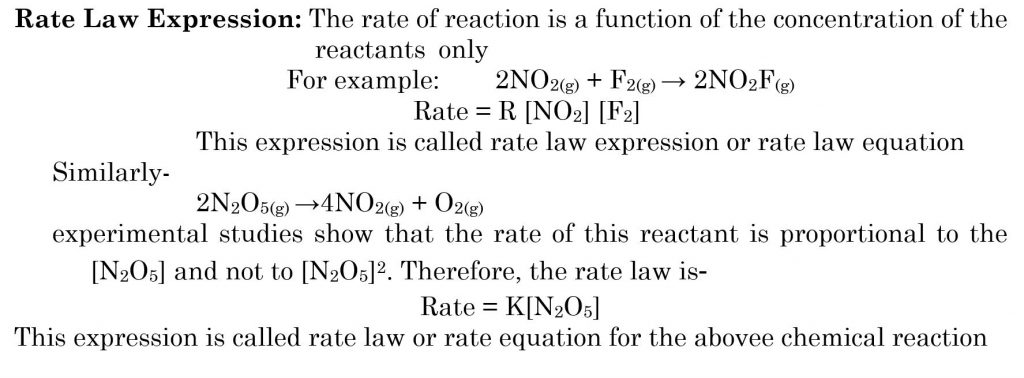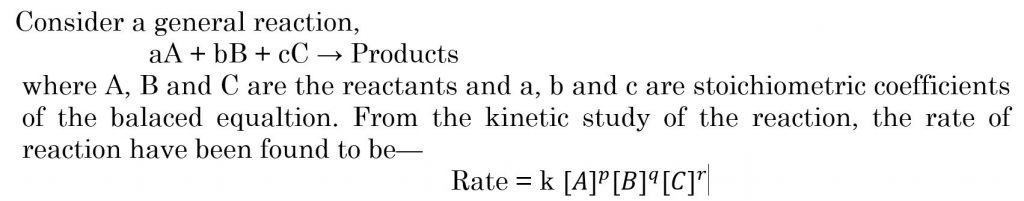where p, q, and r are constant numbers or the power of the concentration of the reactant A, B and C respectively, on which the rate of reactant depends
—The values p, q and r are determined experimentally and may or may not be equal to a, b and c
—The mathemical expression which denotes the experimentally observed rate of a reaction in terms of the concerntrations of the reacting species which influences the rate of reactant is called rate law or rate equation.
— The power of concentration of reactnts in rate law expession may or may not be same as the coeefucient of reactant in the balanced chemical equation.
— On the other hand., the law of mass action given the rate expression on the basis of overall balanced equation.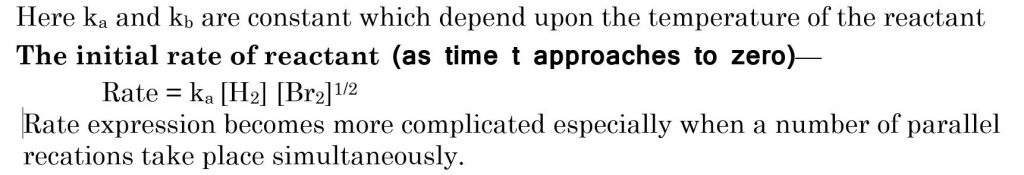All the topics studied under Chemical Kinetics are very useful for CSIR NET coaching in Chemistry, GATE coaching in Chemistry and IIT JAM coaching in Chemistry.